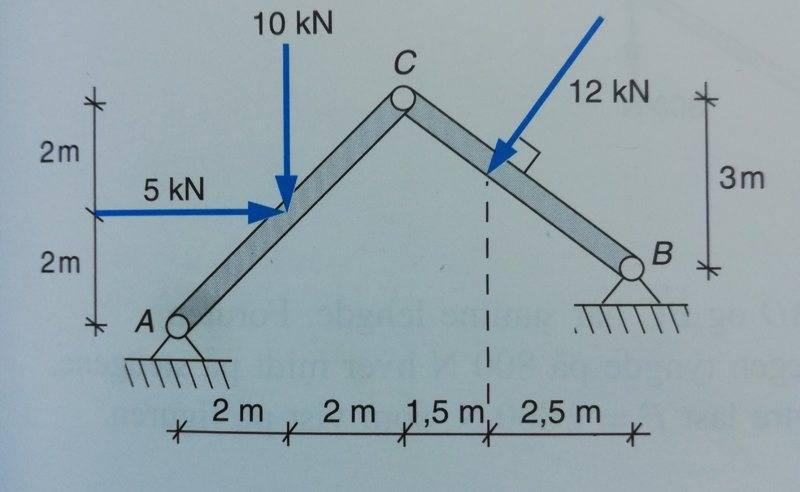# Statics and equilibrium - asking for some guidance

Thread moved from the technical forums, so no Homework Template is shown
Hi,

I'm not used to communicate physics in English, so my language may be a bit off.

This task is fairly simplistic. It's a figure which is held up in points A and B (both having an x and y force exerted on them), and the figure has a joint C. It is depicted in attached files.

The answers are supposed to be F_A = 13,00 kN, F_B = 7,85 kN, F_C = 9,88 kN.

My results are erroneous.

Can anyone please give me some guidance?

Here's my process:

I separate A-C from B-C and get C with internal forces with equally large opposing forces.

Figure A-C:

Sigma M_A (clockwise is +) = 0

5*2 + 10*2 - 4*C_Ay + 4*C_Ax = 0

=> 30 - 4C_Ay + 4C_Ax = 0, this is (1)

Figure B-C:

Sigma M_B (clockwise is +) = 0

(- cos(arctan(3/4)) * 12 * 2,5) - (sin(arctan(3/4)) * 12 * (tan(arctan(3/4)) * 2,5)) - C_Bx * 3 - C_By *4 = 0

- 37,5 - 3C_Bx - 4 C_By = 0, this is (2)

I find an expression for one of the missing values, and put this in the other formula.

And my result is wrong. Always wrong for these tasks. I must do some fundamental error. Any help will be appriciated.#### Attachments

Stephen Tashi
Sigma M_B (clockwise is +) = 0

- 37,5 - 3C_Bx - 4 C_By = 0, this is (2)

Why are you using negative signs in front of C_Bx and C_By?

Isn't the positive x-direction to the right and the positive y-direction upward?

Because C has internal forces and I assumed direction. Both of which work against momentum. On the other figure there's opposite forces in C. Or am I wrong?

Stephen Tashi